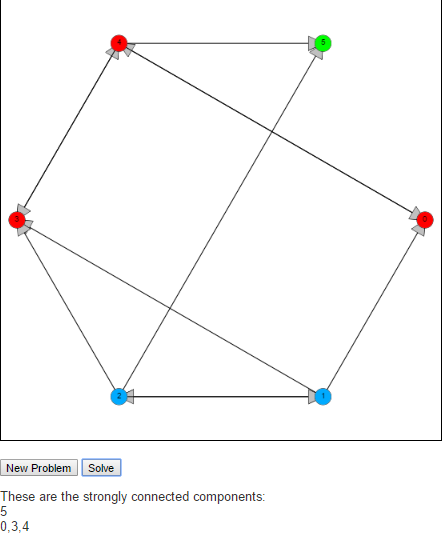Tarjan’s Strongly Connected Components AlgorithmA strongly connected component of a graph is a subgraph S of G where every pair of nodes, u and v in S there is a path from u to v and a path from v to u.

To find these strongly connected components we implement Tarjan’s algorithm. The idea behind Tarjan’s algorithm is to begin by running a depth first search from an arbitrary node in the graph, labeling nodes reachable from this start node in the order they are reached. The algorithm is also interested in the “oldest” node that could be reached by a given node. This is indicated by the keeping track of the lowest label that can be reached from that node. We will call the first property label(v) and the second lowlink(v).

When the algorithm starts label(v) is the same as lowlink(v) whenever a node is discovered. As the algorithm is executed, the DFS is being run on each discovered node, which in turn updates the lowlink(v) property telling of (older) nodes that can be reached. If an older node can be reached, then we update lowlink. If we reach a node that cannot connect to any older nodes after the DFS call, i.e if label(v) is the same a lowlink(v), then this means that this node does not have a path to any node with a lower label. So this node will be the first node of a new strongly connected component.

Feel free to check it out an let me know what you think in the comments below.